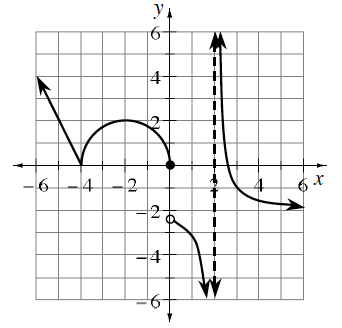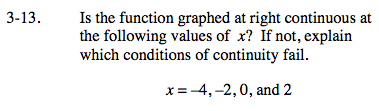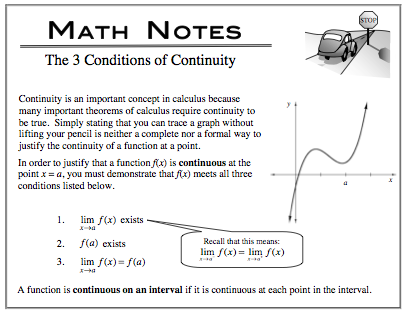### Home > CALC > Chapter Ch3 > Lesson 3.1.1 > Problem3-13

3-13.
1. Is the function graphed below continuous at the following values of x? If not, explain which conditions of continuity fail. Homework Help ✎

x = −4, −2, 0, and 2Now use the three conditions to test if the function is continuous at x = −2, x = 0 and x = 2.

Examine the graph at x = −4

Condition 1:

$\lim_{x\rightarrow -4^{-}}f(x)=0; \ \lim_{x\rightarrow -4^{+}}f(x)=0$

$\lim_{x\rightarrow -4^{-}}f(x)=\lim_{x\rightarrow -4^{+}}f(x).$

$\text{Therefore }\lim_{x\rightarrow -4}f(x)\text{ exists.}$

Condition 2:

f(-4) = 0.
Therefore f(−4) exists.

Condition 3:

$\lim_{x\rightarrow -4}f(x)=f(-4).$

f(x) is continuous at x = −4